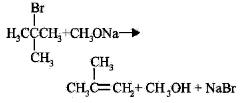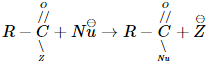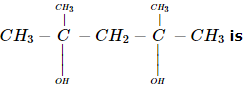Courses

# Sample AFMC Chemistry Mock Test

## 50 Questions MCQ Test AFMC Mock Test Series 2021 | Sample AFMC Chemistry Mock Test

Description
This mock test of Sample AFMC Chemistry Mock Test for NEET helps you for every NEET entrance exam. This contains 50 Multiple Choice Questions for NEET Sample AFMC Chemistry Mock Test (mcq) to study with solutions a complete question bank. The solved questions answers in this Sample AFMC Chemistry Mock Test quiz give you a good mix of easy questions and tough questions. NEET students definitely take this Sample AFMC Chemistry Mock Test exercise for a better result in the exam. You can find other Sample AFMC Chemistry Mock Test extra questions, long questions & short questions for NEET on EduRev as well by searching above.
QUESTION: 1

### Which of the following will not be soluble in sodium hydrogen carbonate ?

Solution:

The reaction is as follows:

Acid + NaHCO3 Sodium salt of acid((soluble) + H2CO3

Among all the given compounds, o-nitrophenol is weaker acid than HCO3Hence, it does not react with NaHCO3.

QUESTION: 2

### The segment of DNA which acts as the instrumental manual for the synthesis of protein is

Solution:

The segment of DNA Which acts as the instrumental manual for the synthesis of the protein is a gene. Every protein in a cell has a corresponding gene.

QUESTION: 3

### Reaction of tert-butyl bromide with sodium methoxide produces

Solution:

Isobutylene is obtained.Thus, the reaction produces isobutylene.

QUESTION: 4

A neutral atom (At. no. > 1) has

Solution:
QUESTION: 5

A carboxylic acid is converted into its anhydride using

Solution: This white crystalline solid is the anhydride of phosphoric acid. It is a powerful desiccant and dehydrating agent.The direct reaction of a carboxylic acid with an amine would be expected to be difficult because the basic amine would deprotonate the carboxylic acid to form a highly unreactive carboxylate. However when the ammonium carboxylate salt is heated to a temperature above 100 degreeC water is driven off and an amide is formed.
QUESTION: 6

Enzymes

Solution:
QUESTION: 7

The iso-electronic pair is

Solution:
QUESTION: 8

The geometric form of crystals is the result of orderly arrangement of

Solution:
QUESTION: 9

The rate of the reactionis fastest when Z is

Solution:
QUESTION: 10

Just before attaining equilibrium by a reversible reaction, it is found that

Solution:
QUESTION: 11

For the reaction 2A + B → 3C + D. Which of the following does not express the reaction rate?

Solution:
QUESTION: 12

Which of the following favours the backward reaction in a chemical equilibrium?

Solution: If the concentration of one or more products is increased then according to Le-chat Lier's principle the system will try to minimise the change i.e., the system will try to decrease the concentration of products and as such the reaction will proceed in backward direction. Thus backward reaction is favoured.
QUESTION: 13

Effect of temperature on rate of reaction given by

Solution:
QUESTION: 14

On Pauling scale which of the following does not have electronegativity ≥ 3.0

Solution:
QUESTION: 15

The heat of combustion of methane at 298K is expressed by CH4(g) + 2O2(g) → CO2(g) + 2H2O(l), ΔH = -890.2kJ. The magnitude of ΔE of the reaction at this temperature is

Solution:
QUESTION: 16

The additive used in composite propellants is

Solution:
QUESTION: 17

Which of the following electronic configuration corresponds to an inert gas?

Solution:

As the electronic configuration of inert gases is ns2 np6 ,thus the element containing electronic configuration 1s22s22p6 , is an inert gas.

QUESTION: 18

A neutral fertilizer among the following compounds is

Solution:
QUESTION: 19

The IUPAC name forSolution:
QUESTION: 20

In hydrogen-oxygen fuel cell,combustion of hydrogen occurs to

Solution:
QUESTION: 21

At STP 1.12 litre of H2 is obtained on flowing a current for 965 seconds in a solution. The value of current is

Solution:

1.12 L at N.T.P. = 1.12/22.4 mol = 0.05 mol
1 mol of H2 is obtained by passing electricity = 2 x 96500 C
0.05 mol of H2 is obtained by electricity = 2 x 96500 x 0.05
Now if I is current strength,
I x 965 = 2 x 96500 x 0.05 or I = 10 amp

QUESTION: 22

An example of an oxide ore is

Solution:
QUESTION: 23

Alkali metals impart colour to Bunsen flame due to

Solution:
QUESTION: 24

The important oxide ore of iron is

Solution:
QUESTION: 25

Magnesium can be obtained by

Solution:
QUESTION: 26

A hydrocarbon reacts with hypochlorous acid to give 2-chloroethanol. The hydrocarbon is

Solution:
QUESTION: 27

...... test is used for detecting unsaturation in hydrocarbons

Solution: Option D is correct.1% cold KMnO4/OH- (pink coloured solution)is turned into Colourless solution+MnO2(brown ppt)its an important test for unsaturation in hydrocarbons.
QUESTION: 28

Heavy water is used in nuclear reactors as

Solution:
QUESTION: 29

What is the conjugate base of OH⁻?

Solution: When acid give H+ then the remaining of its part is called conjugate base. So if we remove H+ from OH- ,we get O2-. Hence O2- is the conjugate base of OH-. P.S:- OH- is also a conjugate base of H2O.
QUESTION: 30

A radioactive isotope decays at such a rate that after 96 minutes only 1/8 th of the original amount remains. The half-life of this nuclide in minutes is

Solution:
QUESTION: 31

The half life period of a radioactive element is 140 days. After 560 days, one gram of the element will deduce to

Solution:
QUESTION: 32

Ethyl bromide on treatment with alcoholic KOH gives

Solution:
QUESTION: 33

The isotope C-14 is

Solution:
QUESTION: 34

Which of the following is employed as Tranquilizer?

Solution:
QUESTION: 35

The first ionisation potential of Group 13 elements is lower than that of Group 2 elements, because

Solution:
QUESTION: 36

Which one of the following is a typical example of compound which exists as zwitter ion?

Solution: Since, glycine is an amino acid which has tend. to form zwitter in in water hence among the given option only (c) is correct
QUESTION: 37

Aluminium chloride exists as

Solution: Al has 3 only valance electrons & therefore forms single covalent bonds with Cl atoms. but AlCl3 leaves the Al atom with only 6 electrons in its outer shell. Each AlCl3 molecule contains 3 Cl atoms that have lone pairs of electrons uninvolved in bonding. So, a dative or co-ordinate bond forms from a Cl atom on 1 molecule to the other. Consequently, the molecule dimerise so that each Al atom has share of 8 electrons in its outer shell. So it exists as dimer.....
QUESTION: 38

The liquified metal expanding on solidification is

Solution:
QUESTION: 39

Cellulose acetate is a

Solution:
QUESTION: 40

Pure N₂ gas is obtained from

Solution:
QUESTION: 41

C₂H₆(g) + nO₂ → CO₂ (g) + H₂O (l) In this equation, the ratio of the coefficients of Co₂ and H₂O is

Solution:
QUESTION: 42

Synthetic human hair wigs are made from a copolymer of vinyl chloride and acrylonitrile, and is called

Solution: Dynel is a synthetic fabric characterised by it's strength & high resistance to heat & burning hence used in making hair wigs etc.
QUESTION: 43

How many grams of CH₃OH would have to be added to water to prepare 150 ml a solution that is 2.0 M CH₃OH ?

Solution:
QUESTION: 44

10 ml of conc. H₂SO₄ (10 molar) is diluted to 1 litre. The approximate strength of dilute acid could be

Solution:
QUESTION: 45

In which of the following 8 : 8 type of packing particles is present?

Solution:
QUESTION: 46

According to the kinetic theory of gases

Solution:

All molecules of an ideal gas show random motion. They collide with each other and walls of container during which they lose or gain energy so they may not have same kinetic energy always.

QUESTION: 47

The cause of Brownian movement is

Solution:
QUESTION: 48

Which of the following types of metals make the most efficient catalysts?

Solution:
QUESTION: 49

Zinc amd mercury do not show variable valency like d-block elements because

Solution:
QUESTION: 50

Iron, once dipped in concentrated H₂SO₄, does not displace copper from copper sulphate solution, because

Solution: